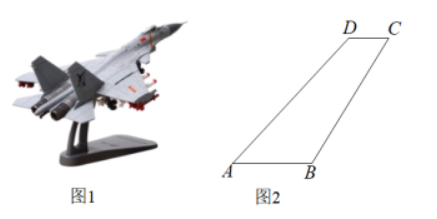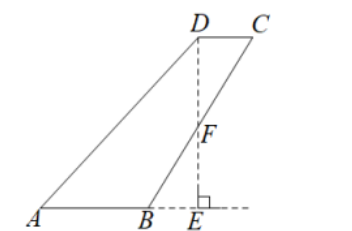【答案】 解: 过 $D$ 作 $D E$ 垂直 $A B$ 的延长线于 $E$ ，交 $B C$ 于点 $F$.
\begin{aligned} & \because A B / / C D, \\ & \therefore D E \perp C D, \\ & \therefore \angle F E B=\angle F D C=90^{\circ}, \end{aligned}

\begin{aligned} & \therefore \angle C F D=30^{\circ}, C F=4 ， D F=2 \sqrt{3} ， \\ & \because B C=8 ， \\ & \therefore B F=4 \text { ， } \\ & \therefore B F=C F . \\ & \text { 在 } \triangle F E B \text { 和 } \triangle F D C \text { 中， }\left\{\begin{array}{l} \angle F E B=\angle F D C \\ \angle F F=C F \\ B F=\angle B F E ， \end{array}\right. \\ & \therefore \triangle F E B \cong \triangle F D C(A A S) . \\ & \therefore B E=C D=2 ， D F=E F=2 \sqrt{3} ， \\ & \because \angle A D C=135^{\circ}, \angle F D C=90^{\circ}, \\ & \therefore \angle A D E=45^{\circ}, \\ & \therefore A E=D E=4 \sqrt{3}, \\ & \therefore S_{A B C D}=S_{\triangle A E D}=\frac{1}{2} A E \cdot D E=\frac{1}{2} \times 4 \sqrt{3} \times 4 \sqrt{3}=24 . \end{aligned}